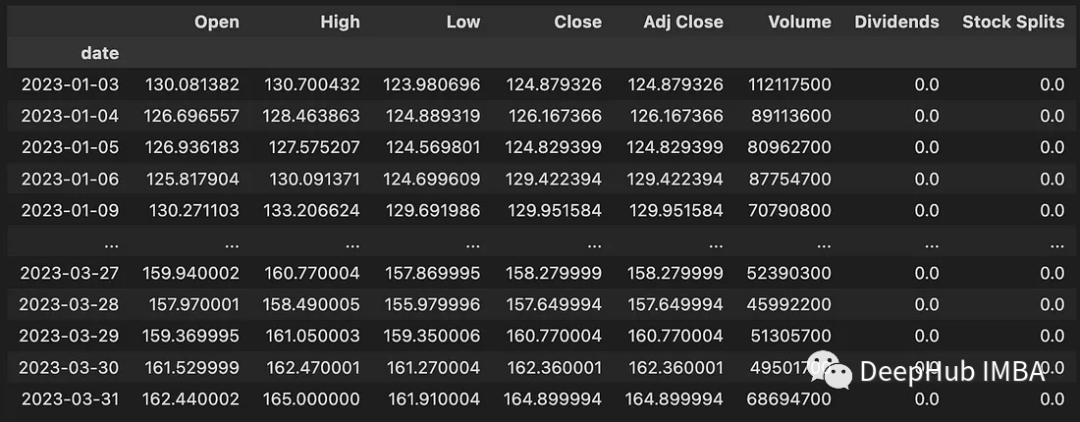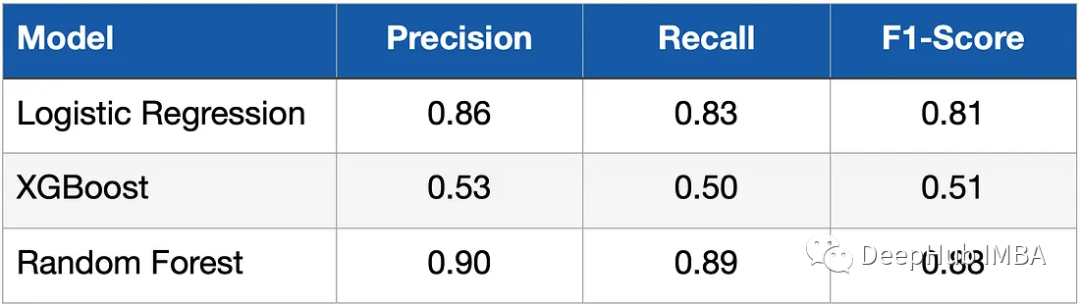# 将时间序列转换为分类问题``  pip install pandas numpy “openbb[all]” swifter scikit-learn``

## 业务理解

``  预测股票代码 AAPL 的股价第二天会上涨还是下跌。``

## 数据理解和准备

`````` data = openbb.stocks.load(
symbol = 'AAPL',
start_date = '2023-01-01',
end_date = '2023-04-01',
monthly = False)
data``````• Open：美元每日开盘价
• High：当日最高价（美元）
• Low：当日最低价（美元）
• Close：美元每日收盘价
• Volume：交易的股票数量
• Dividends：已付股息
• Stock Splits：股票分割执行

`````` defget_training_data(symbol, start_date, end_date, monthly_bool=True, lookback=10):
symbol=symbol,
start_date=start_date,
end_date=end_date,
monthly=monthly_bool)
data=get_label(data)
data_up_down=data['up_down'].to_numpy()
training_data=get_sequence_data(data_up_down, lookback)
returntraining_data``````

`````` defencoding(n):
ifn>0:
return1
else:
return0
defget_label(data):
data['Delta'] =data['Close'] -data['Open']
returndata``````

`````` defget_sequence_data(data_up_down, lookback):
shape= (data_up_down.shape -lookback+1, lookback)
strides=data_up_down.strides+ (data_up_down.strides[-1],)
returnnp.lib.stride_tricks.as_strided(data_up_down, shape=shape, strides=strides)``````

`` get_sequence_data(np.array([1, 2, 3, 4, 5, 6]), 3)``

`````` array([[1, 2, 3],
[2, 3, 4],
[3, 4, 5],
[4, 5, 6]])``````

`````` data = get_training_data(symbol = 'AAPL', start_date = '2023-01-01', end_date = '2023-04-01', monthly_bool = False, lookback=10)
pd.DataFrame(data).to_csv("data/data_aapl.csv")``````

## 建模

`````` data = pandas.read_csv("./data/data_aapl.csv")
X=data.iloc[:,:-1]
Y=data.iloc[:,-1]
X_train, X_test, y_train, y_test = train_test_split(X, Y, test_size=0.33, random_state=4284, stratify=Y)``````

`````` model_lr = LogisticRegression(random_state = 42)
model_lr.fit(X_train,y_train)
y_pred = model_lr.predict(X_test)``````

XGBoost：

XGBoost 是为速度和性能而设计的梯度提升决策树的实现。它属于树提升算法，将许多弱树分类器依次连接。

`````` model_xgb = XGBClassifier(random_state = 42)
model_xgb.fit(X_train, y_train)
y_pred = model_xgb.predict(X_test)``````

`````` model_rf = RandomForestClassifier(random_state = 42)
model_rf.fit(X_train, y_train)
y_pred = model_rf.predict(X_test)``````

## 评估## 总结

https://avoid.overfit.cn/post/57a12ff0cf964fbebf1b27bc72fb2bbb